Courses

# Three Hinged Arch (Part - 1) Civil Engineering (CE) Notes | EduRev

## Civil Engineering (CE) : Three Hinged Arch (Part - 1) Civil Engineering (CE) Notes | EduRev

The document Three Hinged Arch (Part - 1) Civil Engineering (CE) Notes | EduRev is a part of the Civil Engineering (CE) Course Structural Analysis.
All you need of Civil Engineering (CE) at this link: Civil Engineering (CE)

Instructional Objectives:

After reading this chapter the student will be able to

1. Define an arch.
2. Identify three-hinged, two-hinged and hingeless arches.
3. State advantages of arch construction.
4. Analyse three-hinged arch.
5. Evaluate horizontal thrust in three-hinged arch.

Introduction

In case of beams supporting uniformly distributed load, the maximum bending moment increases with the square of the span and hence they become uneconomical for long span structures. In such situations arches could be advantageously employed, as they would develop horizontal reactions, which in turn reduce the design bending moment.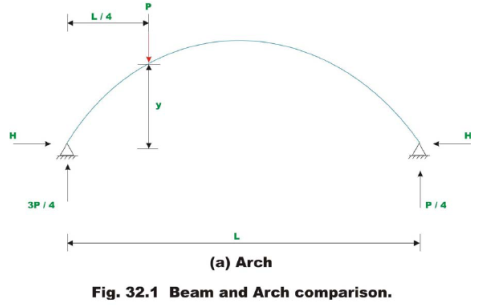For example, in the case of a simply supported beam shown in Fig. 32.1, the bending moment below the load is 3PL/16 . Now consider a two hinged symmetrical arch of the same span and subjected to similar loading as that of simply supported beam. The vertical reaction could be calculated by equations of statics. The horizontal reaction is determined by the method of least work. Now the bending moment below the load is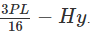It is clear that the bending moment below the load is reduced in the case of an arch as compared to a simply supported beam. It is observed in the last lesson that, the cable takes the shape of the loading and this shape is termed as funicular shape. If an arch were constructed in an inverted funicular shape then it would be subjected to only compression for those loadings for which its shape is inverted funicular.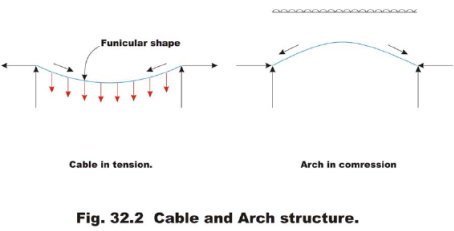Since in practice, the actual shape of the arch differs from the inverted funicular shape or the loading differs from the one for which the arch is an inverted funicular, arches are also subjected to bending moment in addition to compression. As arches are subjected to compression, it must be designed to resist buckling.

Until the beginning of the 20th century, arches and vaults were commonly used to span between walls, piers or other supports. Now, arches are mainly used in bridge construction and doorways. In earlier days arches were constructed using stones and bricks. In modern times they are being constructed of reinforced concrete and steel.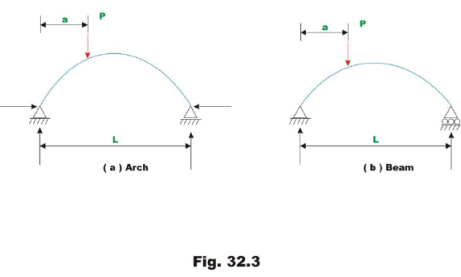A structure is classified as an arch not based on its shape but the way it supports the lateral load. Arches support load primarily in compression. For example in Fig 32.3b, no horizontal reaction is developed. Consequently bending moment is not reduced. It is important to appreciate the point that the definition of an arch is a structural one, not geometrical.

Type of arches

There are mainly three types of arches that are commonly used in practice: three hinged arch, two-hinged arch and fixed-fixed arch. Three-hinged arch is statically determinate structure and its reactions / internal forces are evaluated by static equations of equilibrium. Two-hinged arch and fixed-fixed arch are statically indeterminate structures. The indeterminate reactions are determined by the method of least work or by the flexibility matrix method. In this lesson threehinged arch is discussed.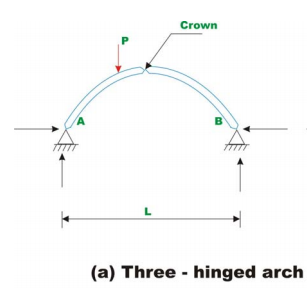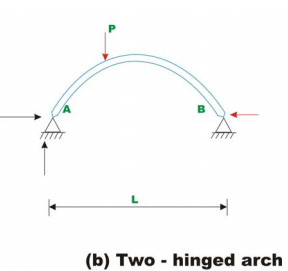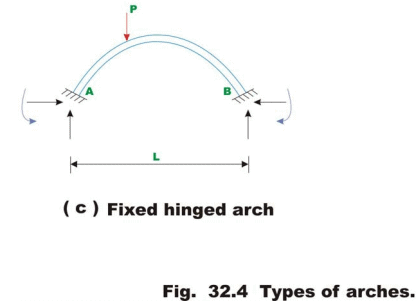Analysis of three-hinged arch

In the case of three-hinged arch, we have three hinges: two at the support and one at the crown thus making it statically determinate structure. Consider a three hinged arch subjected to a concentrated force P as shown in Fig 32.5.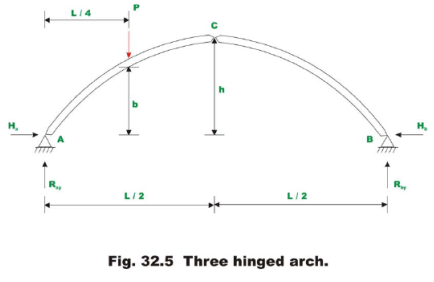There are four reaction components in the three-hinged arch. One more equation is required in addition to three equations of static equilibrium for evaluating the four reaction components. Taking moment about the hinge of all the forces acting on either side of the hinge can set up the required equation. Taking moment of all the forces about hinge A , yields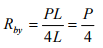(32.1)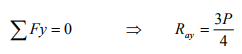(32.2)

Taking moment of all forces right of hinge C about hinge C leads to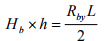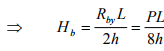(32.3)

Applying ∑Fx = 0 to the whole structure gives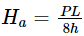Now moment below the load is given by ,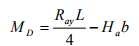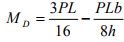(32.4)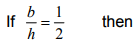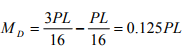(32.5)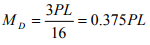(32.6)

For the particular case considered here, the arch construction has reduced the moment by 66.66 %.

Example 32.1

A three-hinged parabolic arch of uniform cross section has a span of 60 m and a rise of 10 m. It is subjected to uniformly distributed load of intensity 10 kN/m as shown in Fig. 32.6 Show that the bending moment is zero at any cross section of the arch.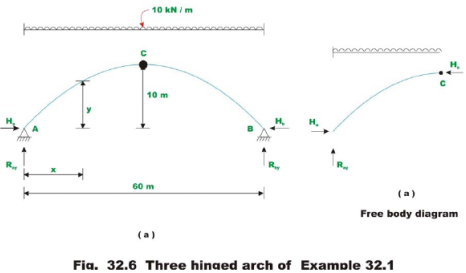Reactions:

Taking moment of all the forces about hinge A , yields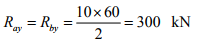(1)

Taking moment of forces left of hinge C aboutC , one gets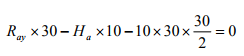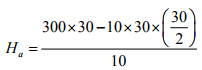(2)

= 450 kN

From ∑Fx = 0 one could write, Hb = 450 kN . The shear force at the mid span is zero.

Bending moment

The bending moment at any section x from the left end is,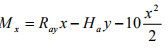(3)

The equation of the three-hinged parabolic arch is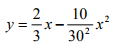(4)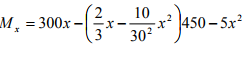= 300x - 300x + 5x2 - 5x2 = 0

In other words a three hinged parabolic arch subjected to uniformly distributed load is not subjected to bending moment at any cross section. It supports the load in pure compression. Can you explain why the moment is zero at all points in a three-hinged parabolic arch?

Offer running on EduRev: Apply code STAYHOME200 to get INR 200 off on our premium plan EduRev Infinity!

## Structural Analysis

30 videos|122 docs|28 tests

,

,

,

,

,

,

,

,

,

,

,

,

,

,

,

,

,

,

,

,

,

;